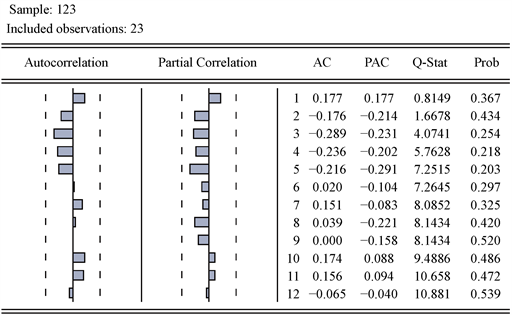﻿ 多项式趋势曲线分析及其在白条猪价格指数中的应用 Analysis of Polynomial Trend Curve and Its Application in Chinese Pig Price Index

Pure Mathematics
Vol. 09  No. 08 ( 2019 ), Article ID: 32406 , 8 pages
10.12677/PM.2019.98111

Analysis of Polynomial Trend Curve and Its Application in Chinese Pig Price Index

Ling Lan, Kai Zuo*

School of Mathematics, Chengdu Normal University, Chengdu SichuanReceived: Sep. 9th, 2019; accepted: Sep. 22nd, 2019; published: Sep. 29th, 2019ABSTRACT

The polynomial trend curve is a very important model to describe the trend of time series, which is widely used in agricultural and industry, environmental and energy, and other fields. In this paper, the mathematical expressions of polynomial model parameters are given by using local summation method, and the time series analysis theory is used to analyze whether the model residual errors are white noise series to establish the corresponding autoregressive moving average model. On the basis of these discussions and with the help of MATLAB 2013b software and EViews 8.0software, taking the Chinese pig price index as an example, the steps of determining the parameters of the polynomial trend model, establishing the model, solving the model and error analysis are all shown in details.

Keywords:Polynomial Trend Curve, Local Summation Method, White Noise Test, Chinese Pig Price Index1. 引言

2. 多项式趋势曲线

2.1. 模型参数的确定

$x\left(k\right)={a}_{0}+{a}_{1}k+{a}_{2}{k}^{2}+\cdots +{a}_{p-1}{k}^{p-1}+{a}_{p}{k}^{p}$ (1)

${S}_{i}=\underset{k=\left(i-1\right)m+1}{\overset{im}{\sum }}x\left(k\right),\text{\hspace{0.17em}}i=1,2,\cdots ,p+1$ . (2)

$\left\{\begin{array}{l}{S}_{1}=\underset{k=1}{\overset{m}{\sum }}x\left(k\right)={a}_{0}m+{a}_{1}\underset{k=1}{\overset{m}{\sum }}k+\cdots +{a}_{p-1}\underset{k=1}{\overset{m}{\sum }}{k}^{p-1}+{a}_{p}\underset{k=1}{\overset{m}{\sum }}{k}^{p},\\ {S}_{2}=\underset{k=m+1}{\overset{2m}{\sum }}x\left(k\right)={a}_{0}m+{a}_{1}\underset{k=m+1}{\overset{2m}{\sum }}k+\cdots +{a}_{p-1}\underset{k=m+1}{\overset{2m}{\sum }}{k}^{p-1}+{a}_{p}\underset{k=m+1}{\overset{2m}{\sum }}{k}^{p},\\ \text{ }\text{ }\text{ }\text{ }\text{ }\text{ }\text{ }\text{ }⋮\\ {S}_{p+1}=\underset{k=pm+1}{\overset{\left(p+1\right)m}{\sum }}x\left(k\right)={a}_{0}m+{a}_{1}\underset{k=pm+1}{\overset{\left(p+1\right)m}{\sum }}k+\cdots +{a}_{p-1}\underset{k=pm+1}{\overset{\left(p+1\right)m}{\sum }}{k}^{p-1}+{a}_{p}\underset{k=pm+1}{\overset{\left(p+1\right)m}{\sum }}{k}^{p}.\end{array}$ (3)

$\left(\begin{array}{ccccccc}m& \underset{k=1}{\overset{m}{\sum }}k& \underset{k=1}{\overset{m}{\sum }}{k}^{2}& \cdots & \underset{k=1}{\overset{m}{\sum }}{k}^{p-2}& \underset{k=1}{\overset{m}{\sum }}{k}^{p-1}& \underset{k=1}{\overset{m}{\sum }}{k}^{p}\\ m& \underset{k=m+1}{\overset{2m}{\sum }}k& \underset{k=m+1}{\overset{2m}{\sum }}{k}^{2}& \cdots & \underset{k=m+1}{\overset{2m}{\sum }}{k}^{p-2}& \underset{k=m+1}{\overset{2m}{\sum }}{k}^{p-1}& \underset{k=m+1}{\overset{2m}{\sum }}{k}^{p}\\ m& \underset{k=2m+1}{\overset{3m}{\sum }}k& \underset{k=2m+1}{\overset{3m}{\sum }}{k}^{2}& \cdots & \underset{k=2m+1}{\overset{3m}{\sum }}{k}^{p-2}& \underset{k=2m+1}{\overset{3m}{\sum }}{k}^{p-1}& \underset{k=2m+1}{\overset{3m}{\sum }}{k}^{p}\\ ⋮& ⋮& ⋮& \ddots & ⋮& ⋮& ⋮\\ m& \underset{k=\left(p-2\right)m+1}{\overset{\left(p-1\right)m}{\sum }}k& \underset{k=\left(p-2\right)m+1}{\overset{\left(p-1\right)m}{\sum }}{k}^{2}& \cdots & \underset{k=\left(p-2\right)m+1}{\overset{\left(p-1\right)m}{\sum }}{k}^{p-2}& \underset{k=\left(p-2\right)m+1}{\overset{\left(p-1\right)m}{\sum }}{k}^{p-1}& \underset{k=\left(p-2\right)m+1}{\overset{\left(p-1\right)m}{\sum }}{k}^{p}\\ m& \underset{k=\left(p-1\right)m+1}{\overset{pm}{\sum }}k& \underset{k=\left(p-1\right)m+1}{\overset{pm}{\sum }}{k}^{2}& \cdots & \underset{k=\left(p-1\right)m+1}{\overset{pm}{\sum }}{k}^{p-2}& \underset{k=\left(p-1\right)m+1}{\overset{pm}{\sum }}{k}^{p-1}& \underset{k=\left(p-1\right)m+1}{\overset{pm}{\sum }}{k}^{p}\\ m& \underset{k=pm+1}{\overset{\left(p+1\right)m}{\sum }}k& \underset{k=pm+1}{\overset{\left(p+1\right)m}{\sum }}{k}^{2}& \cdots & \underset{k=pm+1}{\overset{\left(p+1\right)m}{\sum }}{k}^{p-2}& \underset{k=pm+1}{\overset{\left(p+1\right)m}{\sum }}{k}^{p-1}& \underset{k=pm+1}{\overset{\left(p+1\right)m}{\sum }}{k}^{p}\end{array}\right)\left(\begin{array}{c}{a}_{0}\\ {a}_{1}\\ {a}_{2}\\ ⋮\\ {a}_{p-2}\\ {a}_{p-1}\\ {a}_{p}\end{array}\right)=\left(\begin{array}{c}{S}_{1}\\ {S}_{2}\\ {S}_{3}\\ ⋮\\ {S}_{p-1}\\ {S}_{p}\\ {S}_{p+1}\end{array}\right)$ .(4)

2.2. 计算精度的评估

· 百分比误差(APE)

$\text{APE}\left(k\right)=\frac{x\left(k\right)-\stackrel{^}{x}\left(k\right)}{x\left(k\right)}×100%,k=1,2,\cdots ,h$ . (5)

· 平均绝对百分比误差(MAPE)

$\text{MAPE}=\frac{1}{r-l+1}\underset{k=l}{\overset{r}{\sum }}\frac{|x\left(k\right)-\stackrel{^}{x}\left(k\right)|}{x\left(k\right)}×100%$ , (6)

· 均方根误差(RMSPE)

$\text{RMSPE}=\sqrt{\frac{1}{r-l+1}\underset{k=l}{\overset{r}{\sum }}{\left(\frac{x\left(k\right)-\stackrel{^}{x}\left(k\right)}{x\left(k\right)}\right)}^{2}}×100%$ . (7)

2.3. 残差的白噪声检验及建模

P值法：对于残差序列APE，其样本自相关系数记为 ${\stackrel{^}{\rho }}_{k},k=1,2,\cdots ,r$，r为指定的延迟期数。构造LB统计量

$\text{LB}\left(h,r\right)=h\left(h+2\right)\underset{k=1}{\overset{r}{\sum }}\frac{{\stackrel{^}{\rho }}^{2}{}_{k}}{h-k}~{\chi }^{2}\left(r\right)$ , (8)

${H}_{0}:{\rho }_{1}={\rho }_{2}=\cdots ={\rho }_{r}=0,\forall r\ge 1$ ; ${H}_{1}:\text{ }1\le k\le r,{\rho }_{k}\ne 0,\forall r\ge 1$ . (9)

3. 多项式趋势曲线在白条猪价格指数中的应用

· p = 2时的表达式

$\stackrel{^}{x}\left(k\right)=132.2933+0.2485k+0.0846{k}^{2}$ . (10)

· p = 3时的表达式

$\stackrel{^}{x}\left(k\right)=134.3811-0.7934k+0.2035{k}^{2}-0.0037{k}^{3}$ . (11)

· p = 4时的表达式

$\stackrel{^}{x}\left(k\right)=127.4445+3.9015k-0.7084{k}^{2}+0.0634{k}^{3}-0.0016{k}^{4}$ . (12)Table 2. Computation results of three polynomial trend curves (2019)Figure 1. Sequence diagram of residual APEFigure 2. APE correlation coefficient diagram

$\stackrel{^}{x}\left(k\right)=134.3811-0.7934k+0.2035{k}^{2}-0.0037{k}^{3}$ . (13)Table 3. Prediction results of trend curves of third-order polynomials

4. 结束语

Analysis of Polynomial Trend Curve and Its Application in Chinese Pig Price Index[J]. 理论数学, 2019, 09(08): 849-856. https://doi.org/10.12677/PM.2019.98111

1. 1. 贾俊平, 何晓群, 金勇进. 统计学(第7版)[M]. 北京: 中国人民大学出版社, 2019.

2. 2. 宋廷山, 王坚, 刁艳华, 郭思亮. 应用统计学——以EXCEL为分析工具(第2版)[M]. 北京: 清华大学出版社, 2018.

3. 3. 周永道, 王会琦, 吕王勇. 时间序列分析及应用[M]. 北京: 高等教育出版社, 2016.

4. 4. 刘田, 谈进. 基于局部多项式回归去势的非线性趋势序列的单位根检验[J]. 数量经济技术经济研究, 2012(8): 137-149.

5. 5. 沈松英, 杨立刚, 杨斌. 广东省梅毒报告发病率曲线拟合及发病率趋势预测[J]. 中国艾滋病性病, 2011(3): 341-343.

6. 6. 段树乔, 段方婕. 基于多项式回归函数的电网公司售电量预测方法[J]. 数学的实践与认识, 2012, 42(18): 76-81.

7. 7. 高峰, 余志豪. 基于二次多项式趋势曲线预测模型的农业生产用电市场研究——以浙西地区进行实证分析[J]. 南京工程学院学报(社会科学版), 2015, 15(4): 25-29.

8. 8. 左凯, 李虎, 张玉林, 吴文青. 一种确定k阶趋势曲线参数的新方法[J]. 应用数学进展, 2019(8): 1384-1391.

9. 9. Wu, L.F., Liu, S.F. and Yang, Y.J. (2016) Grey Double Exponential Smoothing Model and Its Application on Pig Forecasting in China. Applied Soft Computing, 39, 117-123. https://doi.org/10.1016/j.asoc.2015.09.054

10. 10. Zhou, W.J. and Pei, L.L. (2019) The Grey Generalized Verhulst Model and Its Application for Forecasting Chinese Pig Price Index. Soft Computing, 1-14.

11. NOTES

*通讯作者。